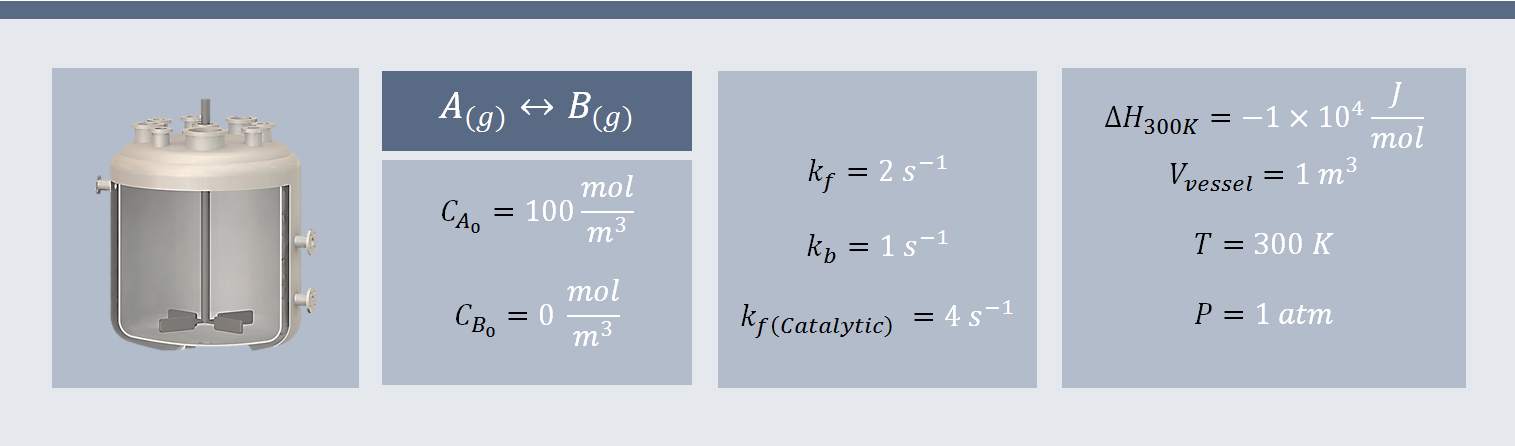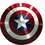# A Problem On Equilibrium Constant

Le Chateliers principle tells us what will happen when equilibrium is disturbed qualitatively. This numerical problem is designed to explain the same principle quantitatively.This problem is large and its very purpose is to clear doubts regarding chemical equilibrium.

Consider a elementary reaction taking place in a closed vessel of volume $1 m^3$ $A_{(g)} \rightleftharpoons B_{(g)}$ We start with pure A with initial concentration of 100 $mol/m^3$.Reaction temperature and pressure are 300 K and 1 atm respectively. Other details are shown below.To attack this problem, we write expression for equilibrium concentration $K_c$.

$K_c=\frac{C_{be}}{C_{ae}}$

$K_c=\frac{k_f}{k_b}=\frac{2}{1}=2$

$\frac{C_{be}}{C_{ae}}=2$

To solve for $C_{ae}$, we need to express $C_{be}$ in terms of $C_{ae}$.This can be done as follow

$C_{be}=C_{a0}-C_{ae}$

$\frac{C_{a0}-C_{ae}}{C_{ae}}=2$

Solving for $C_{ae}$

$C_{ae}=33.33 \space mol/m^3$

$C_{be}=100-33.33=66.67 \space mol/m^3$

If this reaction would be irreversible,we would have 0 mol of A and 100 mol of B.Remember Van't Hoff equation! That will give equilibrium constant at a particular temperature .For this, we need to solve following differential equation.

$\frac{d(\ln K_c)}{dT}=\frac{\Delta H}{RT^2}$

Separating variables,

$d(\ln K_c)=\frac{\Delta H}{R}\frac{dT}{T^2}$

And integrating gives

$\ln \frac{K_2}{K_1}=\frac{\Delta H}{R}(\frac{1}{T_1}-\frac{1}{T_2})$

We will use this relation to find $K_c$ at T=273K.

$\Delta H=-10^4$

$K_1=2\quad at \quad T_1=300 K$

$K_2=?\quad at \quad T_2=273 K$

This will give $K_2=2.97$.

From equilibrium constant formula

$\frac{C_{a0}-C_{ae}}{C_{ae}}=2.97$

$C_{ae}=25.17\space mol/m^3$

And $C_{be}=100-25.17=74.83\space mol/m^3$

We see that $C_{be}$ is increased when temperature is lowered.

Similarly one can calculate $K_c$ at T=323 K

$K_{323}=1.50$

$C_{ae}=39.95\space mol/m^3$

And $C_{be}=100-39.95=60.05\space mol/m^3$

We notice that $C_{be}$ is increased when temperature is lowered.

These results prove Le chatelier principle :A exothermic reaction is favoured at low temperature as we get equilibrium concentration of B increases from 66.67 $mol/m^3$ to 74.83 $mol/m^3$ when temperature is lowered from 300K to 273K.We know at T=300 K, $C_{ae}=33.33\space mol/m^3$

If we can relate concentration of A with time, problem can be easily solved by finding t for this $C_{ae}$.

For this we proceed as follow.

Material balance for A:

Rate of accumulation of A=input-output+generation-consumption

Since reaction is taking place in closed vessel,both input=0 and output=0

Rate of accumulation of A=$\frac{dC_a}{dt}$

Generation=$k_bC_b$

Consumption=$k_fC_a$

Putting these terms in matrial balance equation gives

$\frac{dC_a}{dt}=k_bC_b-k_fC_a$

Writing $C_b$ in terms of $C_a$

$C_{be}=C_{a0}-C_{ae}$

$\frac{dC_a}{dt}=k_b(C_{a0}-C_{ae})-k_fC_a$

$\frac{dC_a}{dt}=k_bC_{a0}-(k_f+k_b)C_a$

$\frac{dC_a}{dt}+(k_f+k_b)C_a=k_bC_{a0}$

Substituting values of $k_f=2 s^{-1}$,$k_b=2 s^{-1}$ and $C_{a0}=100 \space mol/m^3$

$\frac{dC_a}{dt}+3C_a=100$

Above equation can be solved using method of integration factor.

$C_a=\frac{100}{3}+Ne^{3t}$

where N is constant of integration.Its value can be found out by initial condition.

$at \quad t=0,C_a=C_{a0}=100 \space mol/m^3$

Which gives $N=\frac{200}{3}$

$C_a=\frac{100}{3}+\frac{200e^{-3t}}{3}$

Now, we have relation between $C_a$ and t.

To find time required for equilibrium,put $C_{ae}=\frac{100}{3}$

$\frac{100}{3}=\frac{100}{3}(1+2e^{-3t})$

This will give

$e^{-3t}=0$

That is $t=\infty$

This is not good.We did such lengthy calculation and got infinity as result.This may predict that equilibrium cannot be achieved in finite time.But if we find time required for $C_a$ to become 33.34, it will be only $t=3.07 s$.This strange behavior can be explained by nature of function $e^{-3t}$ which become asymptote to the line $C=100/3$.For practical purpose,time required for $C_{ae}=33.34$ is enough.We know a catalyst increases rate of reaction that too without actually get consumed.But here question is will it change equilibrium concentration of A and B.Answer is simply no.It increases both rate constant to the same extent so that ratio of rate constant that is $K_c$ is same as in non-catalytic reaction.For proof see How does catalyst work

For finding time required for equilibrium,we proceed as follow:

We have increased rate constant for both forward and backward reaction in catalytic reaction as

$k_{fc}=4 s^{-1} \space and \space k_{bc}=2 s^{-1}$

Now writing material balance for A,

$\frac{dC_a}{dt}+(k_{fc}+k_{bc})C_a=k_bC_{a0}$

Substituting values of $k_{fc}=2 s^{-1}$,$k_{bc}=2 s^{-1}$ and $C_{a0}=100 \space mol/m^3$

$\frac{dC_a}{dt}+6C_a=200$

Try to solve this equation by integration factor method as we did above to get the final equation as

$C_a=\frac{100}{3}+\frac{200e^{-6t}}{3}$

Putting $C_{ae}=\frac{100}{3}$ and solving for t, we will get same result as we got above in case of non-catalytic reaction That is $t=\infty$.This time we know how to avoid this problem.We proceed to find out time for $C_{a}=33.34$ We get $t=1.56 s$

We see that time required for concentration to reach 33.34 is half of what we get in case of non catalytic reaction.

$C_a=\frac{100}{3}+\frac{200e^{-3t}}{3}$

In general,One can easily show that

Time required will be less in catalytic reaction than non-catalytic reaction for same concentration.Here is the graph of concentration of A in two cases.Since one mole of A gives one mole of B, there is no increase or decrease of pressure during reaction.Hence changing pressure at equilibrium will not change equilibrium concentration of A and B.At equilibrium, we have 33.33 mol of A and 66.67 mol of B.Now we are adding another 50 mol of A.How this will disturb the equilibrium.Adding this extra A at previous equilibrium will result in same equilibrium as if it will be if we start with 100+50=150 mol of A.Another way to think is that 50 mol of A will give 33.33 mol B and 16.667 mol A will remain unreacted.So on total we will have 66.67+33.33=100 mol B and 33.33+16.6667=50 mol A.Hence we will have more B at equilibrium.

You can point out that percentage of B in equilibrium mixture is same in both cases(66.67%).But this is not in general.Note by Aamir Faisal Ansari
6 years ago

This discussion board is a place to discuss our Daily Challenges and the math and science related to those challenges. Explanations are more than just a solution — they should explain the steps and thinking strategies that you used to obtain the solution. Comments should further the discussion of math and science.

When posting on Brilliant:

• Use the emojis to react to an explanation, whether you're congratulating a job well done , or just really confused .
• Ask specific questions about the challenge or the steps in somebody's explanation. Well-posed questions can add a lot to the discussion, but posting "I don't understand!" doesn't help anyone.
• Try to contribute something new to the discussion, whether it is an extension, generalization or other idea related to the challenge.

MarkdownAppears as
*italics* or _italics_ italics
**bold** or __bold__ bold
- bulleted- list
• bulleted
• list
1. numbered2. list
1. numbered
2. list
Note: you must add a full line of space before and after lists for them to show up correctly
paragraph 1paragraph 2

paragraph 1

paragraph 2

[example link](https://brilliant.org)example link
> This is a quote
This is a quote
    # I indented these lines
# 4 spaces, and now they show
# up as a code block.

print "hello world"
# I indented these lines
# 4 spaces, and now they show
# up as a code block.

print "hello world"
MathAppears as
Remember to wrap math in $$ ... $$ or $ ... $ to ensure proper formatting.
2 \times 3 $2 \times 3$
2^{34} $2^{34}$
a_{i-1} $a_{i-1}$
\frac{2}{3} $\frac{2}{3}$
\sqrt{2} $\sqrt{2}$
\sum_{i=1}^3 $\sum_{i=1}^3$
\sin \theta $\sin \theta$
\boxed{123} $\boxed{123}$

Sort by:

Feel free to post if you find something wrong in this note.

- 6 years ago